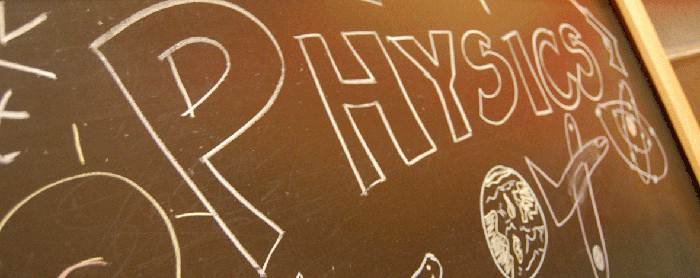Medical Sample papers

Questions on Laws of Motion (Physics)Newton’s Laws of Motion Questions with Answers:

Ques. Which of the following has the least inertia?
(a) Pencil
(b) School bag
(c) Book
(d) Table
Ans. (a)

Ques. If A and B are two objects with masses 10kg and 100kg respectively then
(a) A has more inertia than B
(b) B has more inertia than A
(c) A and B have the same inertia
(d) none of the two has inertia
Ans. (b)

Ques. A passenger in a bus tends to fall backward when the bus starts suddenly due to the
(a) inertia of rest
(b) inertia of motion
(c) inertia of direction
(d) none of the above
Ans. (a)

Ques. A cyclist does not come to rest immediately after he stops peddling due to the
(a) inertia of rest
(b) inertia of motion
(c) inertia of direction
(d) none of these
Ans. (b)

Ques. A rider on a horse back falls forward when the horse suddenly stops. This is due to
(a) inertia of horse
(b) inertia of rider
(c) large weight of the horse
(d) losing of the balance
Ans. (b)

Ques. A force acting on an object of mass 10kg raises its speed from 2m/s to 5m/s in 10seconds. Magnitude of the force is
(a) 3N
(b) 1N
(c) 2N
(d) 5N
Ans. (a)

Ques. Momentum of a gold sphere of radius ‘r’ units is ‘k’ units. Then momentum of another gold sphere of radius ‘2r’ units moving with the same velocity is ________units.
(a) 2k
(b) 4k
(c) 6k
(d) 8k
Ans. (d)

Ques. Two balls of same mass ‘m’ kg and velocity ‘v’ m/s are moving towards each other. What is the total momentum of the system?
(a) 0
(b) –1
(c) –2
(d) 1
Ans. (a)

Ques. A car of mass ‘m’ kg and a truck of mass ‘10m’ kg are moving with the same velocity. The force required to stop the car in a certain distance is ‘F’ N. Then the force required to stop the truck through the same distance is _______ N.
(a) 2F
(b) 5F
(c) 10F
(d) 4F
Ans. (c)

Ques. Action – reaction forces
(a) act on the same body
(b) acts on different bodies
(c) act along different lines
(d) act in the same direction
Ans. (b)

Ques. If action is of 10N, then the reaction will be of
(a) 10N in the same direction
(b) 20N in the same direction
(c) 10N in the opposite direction
(d) 20N in the opposite direction
Ans. (c)

Ques. Swimming is possible on account of
(a) Newton’s first law of motion
(b) Newton’s second law of motion
(c) Newton’s third law of motion
(d) None of these
Ans. (c)

Ques. A car is a rest. We can conclude that the weight caused by gravitational attraction to the Earth and the contact (normal) force of the car with the Earth are equal and opposite because
(a) the two forces are third law pairs.
(b) the net force on the car must be zero.
(c) neither of the above.
(d) all of these
Ans. (b)

Ques. If the two bodies are in contact, the force exists between these two bodies are
(a) gravitational force
(b) electrostatic force
(c) normal force
(d) none of these
Ans. (c)

Ques. Two bodies in contact and if the contact is frictionless the contact force is _____ to the common surface and is known as ______
(a) parallel, frictional force
(b) perpendicular, frictional force
(c) perpendicular force, normal force
(d) parallel, normal force
Ans. (c)

Ques. When a horse pulls a cart, the force that helps the horse to move forward is the force exerted by
(a) the cart on the horse
(b) the ground on the horse
(c) the ground on the cart
(d) the horse on the ground
Ans. (b)

Ques. A lift is moving with a retardation of 5m/s2. The percentage change in weight of person in the lift is (g = 10m/s2)
(a) 100
(b) 25
(c) 50
(d) 75
Ans. (c)

Ques. A spring balance is suspended from a ceiling and another spring balance is attached to the end of the first. A 5kg of mass is hung to lower balance, then
(a) both balance will read 5kg.wt each
(b) the upper reads 5kg.wt & the lower is zero
(c) both of them read 2.5 kg.wt each
Ans. (a)

Ques. The weight of a man in a lift moving upwards is 608N, while the weight of same man in the lift moving downwards with the same acceleration is 380N. His normal weight in newtons is
(a) 488
(b) 588
(c) g
(d) 240
Ans. (a)

Ques. The ratio of the weight of a man is stationary lift and in a lift accelerating downwards with a uniform acceleration ‘a’ is 3:2 the acceleration of the lift is
(a) g/3
(b) g/2
(c) g
(d) 2g
Ans. (a)

Ques. A boat of mass 3000kg, initially at rest is pulled by a force of 1.8 × 104 newtons through a distance of 3metres. Assuming that resistance due to water is neglisible, velocity of the boat is
(a) 4m/s
(b) 6m/s
(c) 8m/s
(d) 10m/s
Ans. (a)

Ques. A man of mass 20kg standing in an elevator whose cable broke suddenly. If the elevator falls freely the force exerted by the floor of the elevator on the mass is
(a) 20N
(b) 10N
(c) 0
(d) none of these
Ans. (c)

Ques. In a game of tug of wars, a condition of equilibrium exists. Both the teams pull the rope with a force of 104N. The tension in the rope is
(a) 104 N
(b) 105 N
(c) 0 N
(d) 2 × 104 N
Ans. (a)

Related: Important MCQs On Biomolecules

Ques. A vehicle of 100kg moving with a velocity of 5m/sec. To stop it in 1/10sec, the required force in opposite direction is
(a) 5000 N
(b) 500 N
(c) 50 N
(d) 1000 N
Ans. (a)